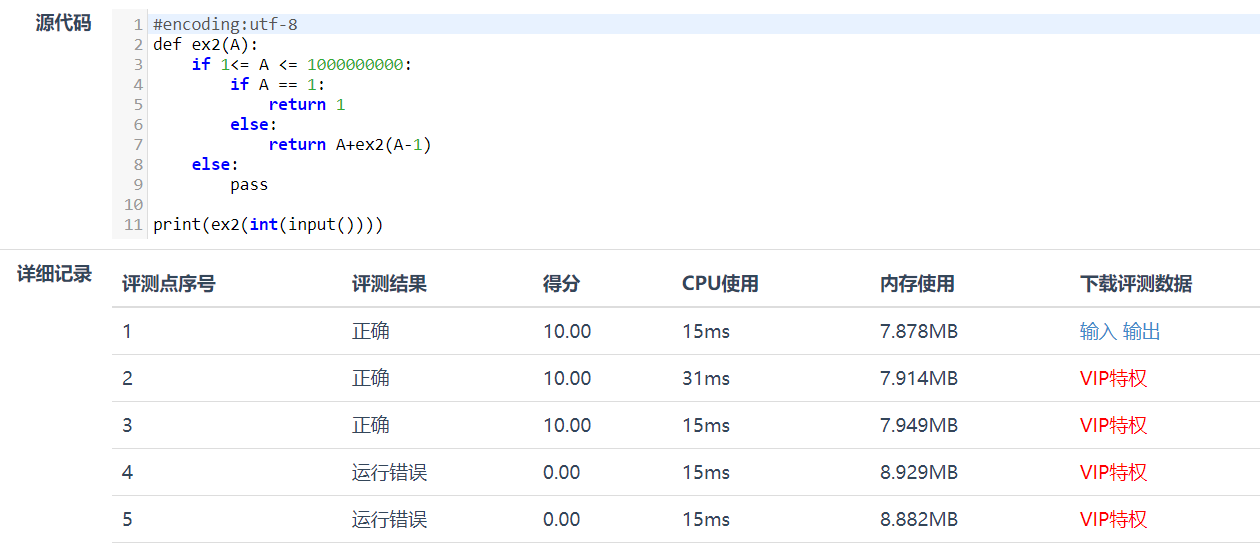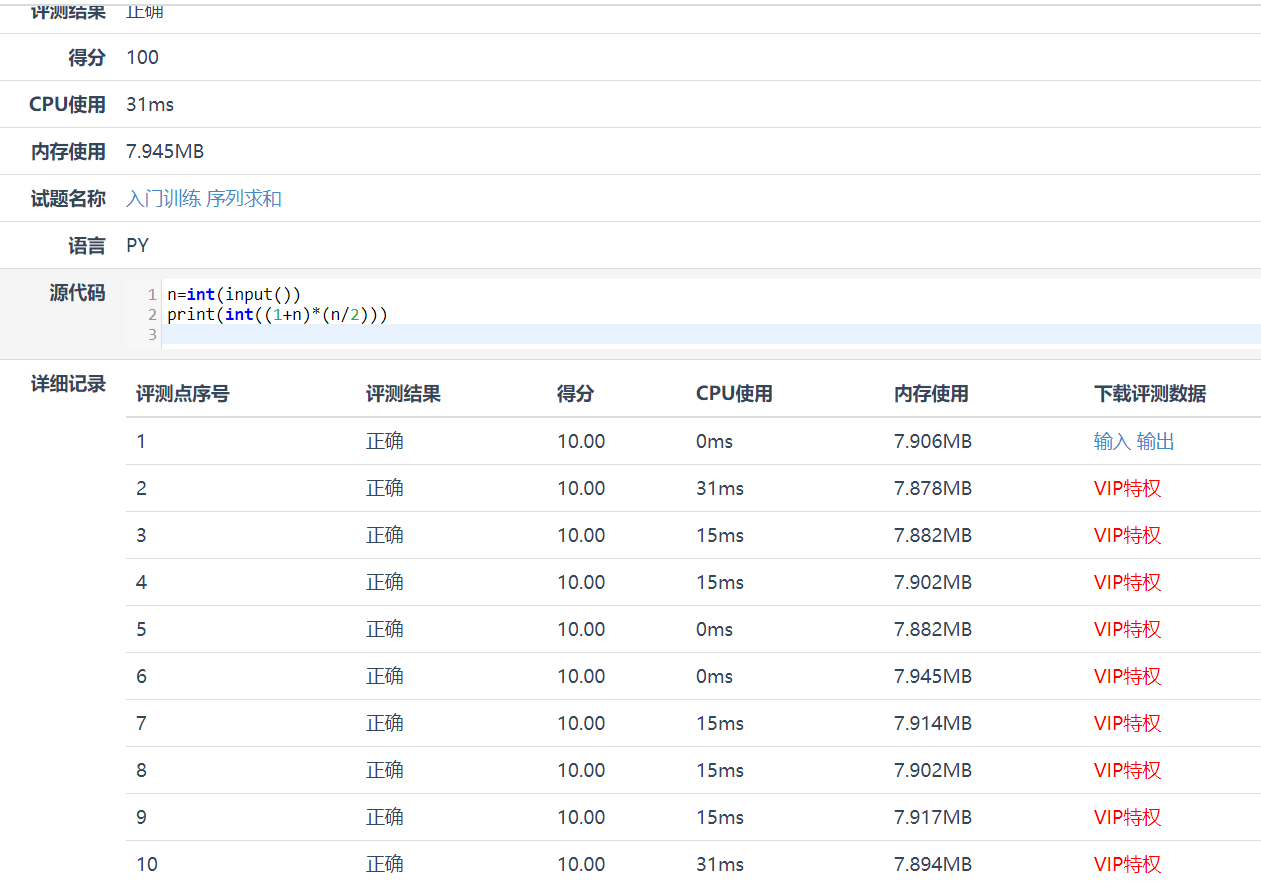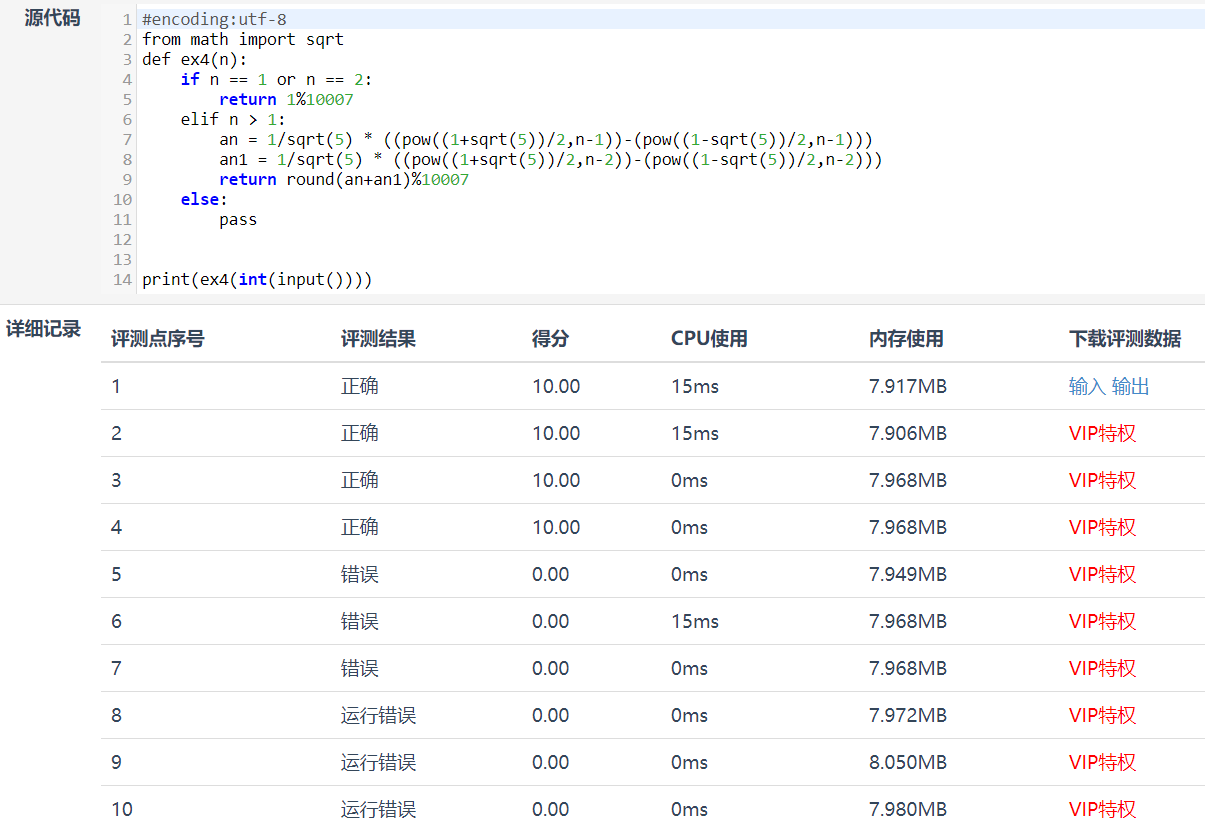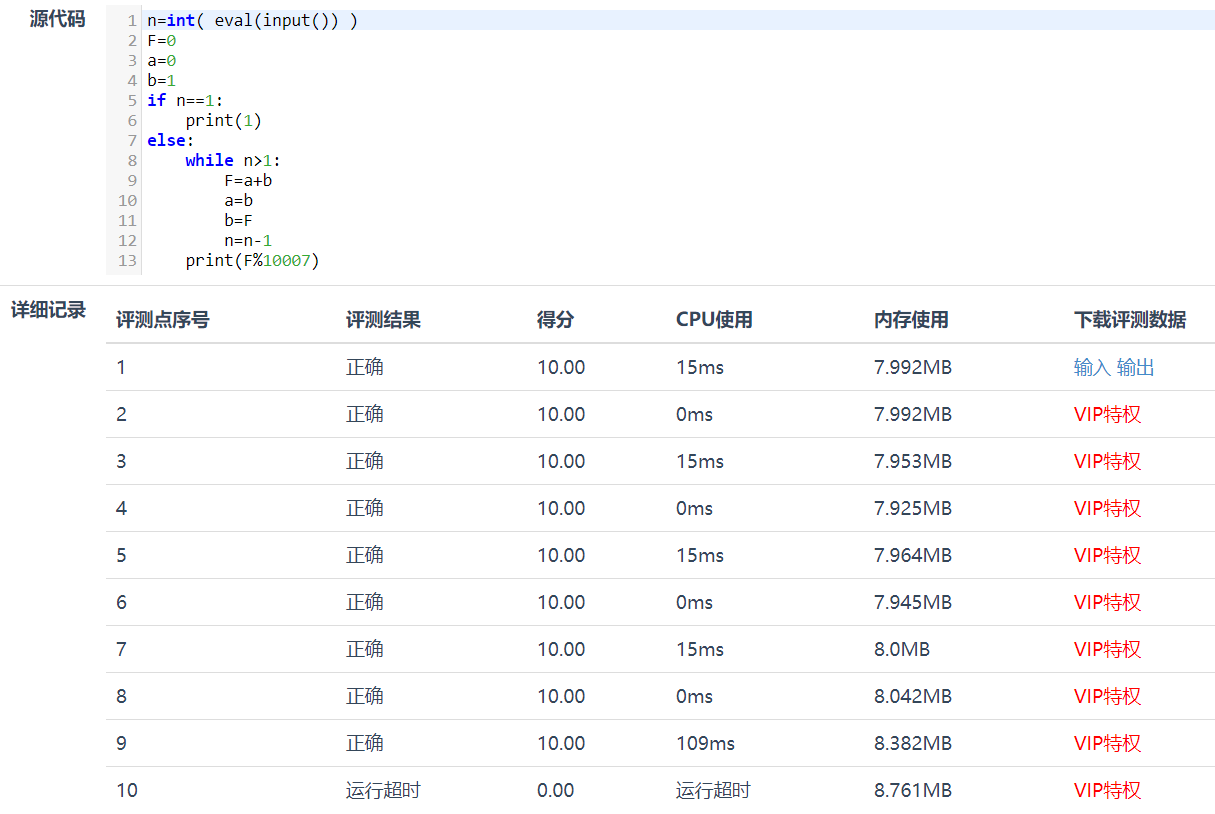## 蓝桥杯Python刷题——入门训练100分评测

2021/2/8 11:44:05 文章标签: 测试文章如有侵权请发送至邮箱809451989@qq.com投诉后文章立即删除

1.AB问题 问题描述 输入A、B&#xff0c;输出AB。 说明&#xff1a;在“问题描述”这部分&#xff0c;会给出试题的意思&#xff0c;以及所要求的目标。 输入格式 输入的第一行包括两个整数&#xff0c;由空格分隔&#xff0c;分别表示A、B。 说明&#xff1a;“输入格式”是描…

1.A+B问题

``````#encoding:utf-8
def ex1():
A,B = (input().split())
A = int(A)
B = int(B)
if A>= -10000 and B<=10000:
return A+B
else:
pass

print(ex1())
``````

2.序列求和``````n=int(input())
print(int((1+n)*(n/2)))
``````3.圆的面积

``````#encoding:utf-8
import math
def ex3(r):
if 1 <= r <= 10000:
return math.pi * r *r
else:
pass

print(format(ex3(int(int(input())*10000000)/10000000),'.7f'))
``````

4.兔子数列问题

Fibonacci数列的递推公式为：Fn=Fn-1+Fn-2，其中F1=F2=1。``````n=int( eval(input()) )
F=0
a=0
b=1
if n==1:
print(1)
else:
while n>1:
F=a+b
b=b%10007
a=b
F=F%10007
b=F
n=n-1
print(F%10007)
``````

暂无相关的数据...